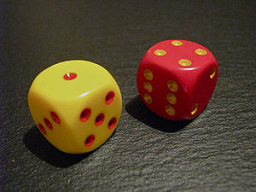# Probability 71784

What is the probability that if you roll the die twice, the sum of 12 will fall?

p =  0.0278

### Step-by-step explanation:Did you find an error or inaccuracy? Feel free to write us. Thank you!

Campo
Dice vs Die:

The word "dice" is plural. So rolling "twice" means you are rolling the pair twice. The probability that one pair will NOT be 12 = 35/36. The probability that you will get at least one 12 in 2 rolls is 1 - (35/36) * (35/36) = .054784

well the first roll result can be anything in case the second roll is 12 and vice versa.
so (1 * 1/36 )*2=0.0556 .

Tips for related online calculators
Need help calculating sum, simplifying, or multiplying fractions? Try our fraction calculator.
Would you like to compute the count of combinations?